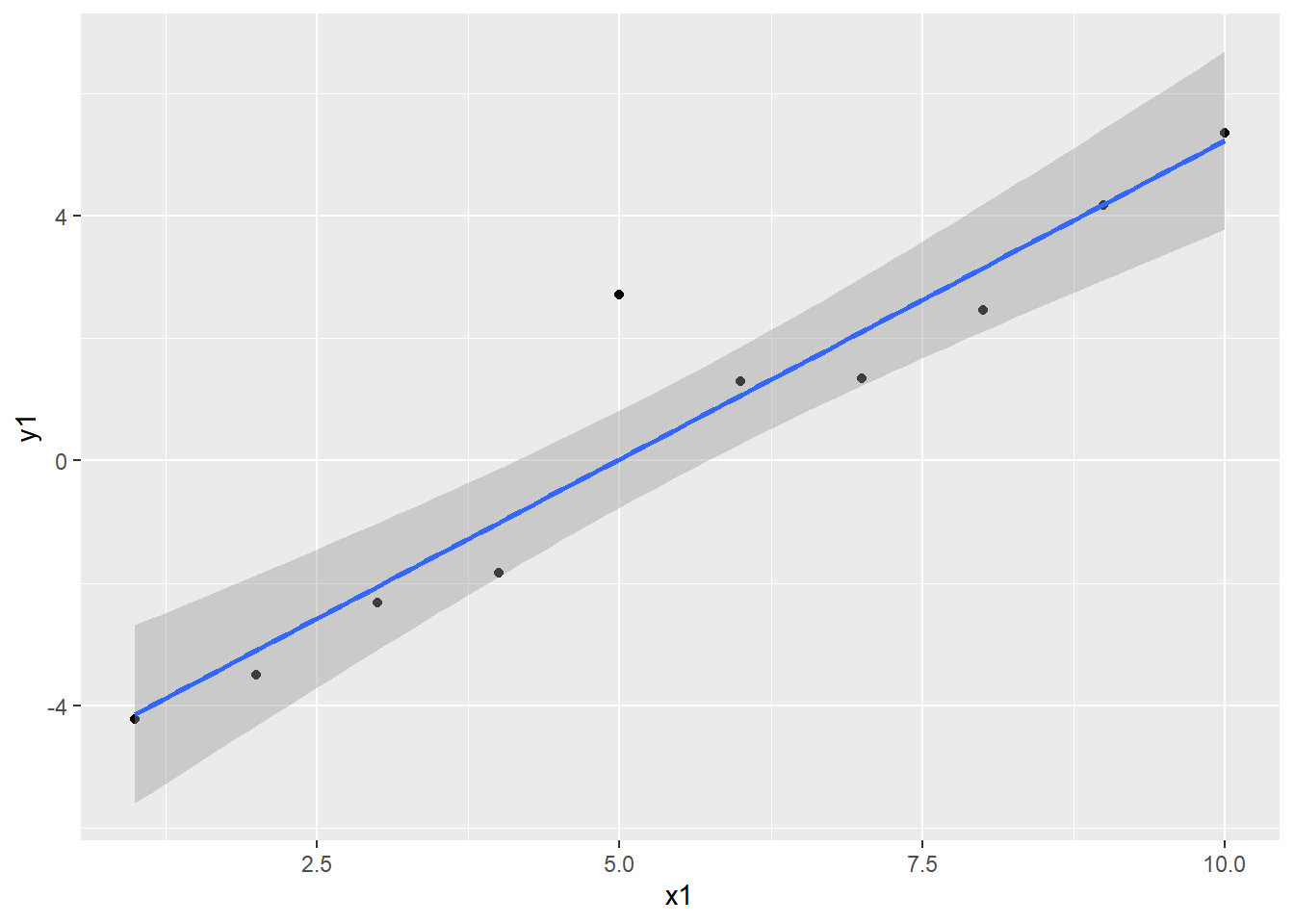# Inverse prediction: terms prediction type

One of the exercises I make Ecological Statistics students do is work backwards from a given value of the dependent variable in a regression to the corresponding values of the independent variable. I usually just get them to eyeball it from a table of predictions. But today a student suggested the “terms” type of prediction might be the answer. Unfortunately I’ve never tried to figure out what type = “terms” actually does1. What is this magic?

library("tidyverse")
df <- tibble(x1 = 1:10,
x2 = rep(1:5, each = 2),
y1 = -5 + 1 * x1 + rnorm(10),
y2 = 0 + 1 * x1 - 1 * x2 + rnorm(10),
y3 = 25 -10 * x1 + 1 * x1^2)
mod1 <- lm(y1~x1, data = df)
mod2 <- lm(y1~x1 + x2, data = df)
mod3 <- lm(y1~x1 + x1^2, data = df)
ggplot(data = df) +
geom_point(mapping = aes(x = x1, y = y1)) +
geom_smooth(mapping = aes(x = x1, y = y1), method = "lm")
## geom_smooth() using formula 'y ~ x'OK, so we’ve got three models, one with a single predictor, one with two predictors, and one with a polynomial model. When there is a single predictor, there is only a single value of the independent variable that gives that value of the dependent variable. In mathematical terms the linear function is “invertible” – you can run it both ways and get a unique answer.

predict(mod1, type = "terms")
##            x1
## 1  -4.6864421
## 2  -3.6450105
## 3  -2.6035789
## 4  -1.5621474
## 5  -0.5207158
## 6   0.5207158
## 7   1.5621474
## 8   2.6035789
## 9   3.6450105
## 10  4.6864421
## attr(,"constant")
##  0.5439744

OK, if that was useful for “inverse prediction” I expect it would be related to the values of x1, but it isn’t. It’s the fitted value (y) plus a constant. A bit of digging online reveals that it is the fitted value for that particular term, after centering the independent variable. The constant is related to the centering process. In all the digging I couldn’t figure out what one uses the terms type for. I’m sure it’s good for something, I just don’t know what! Suggestions in the comments please.

So the question of easily doing inverse prediction is unanswered. Stay tuned for part 2 though, I did learn something in my digging!

1. Code not shown in the post can be found on Github.↩︎